# Final stellation of the icosahedron

Final stellation of the icosahedron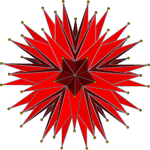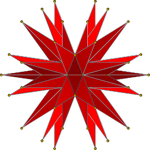Two symmetric orthographic projections
Symmetry groupicosahedral (Ih)
TypeStellated icosahedron, 8th of 59
SymbolsDu Val H
Wenninger: W42
Elements
(As a star polyhedron)
F = 20, E = 90
V = 60 (χ = 10)
Elements
(As a simple polyhedron)
F = 180, E = 270,
V = 92 (χ = 2)
Properties
(As a star polyhedron)
Vertex-transitive, face-transitive
Stellation diagramStellation coreConvex hull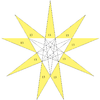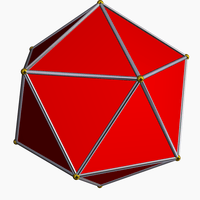Icosahedron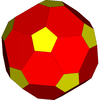truncated icosahedron

In geometry, the complete or final stellation of the icosahedron is the outermost stellation of the icosahedron, and is "complete" and "final" because it includes all of the cells in the icosahedron's stellation diagram.

This polyhedron is the seventeenth stellation of the icosahedron, and given as Wenninger model index 42.

As a geometrical figure, it has two interpretations, described below:

Johannes Kepler researched stellations that create regular star polyhedra (the Kepler-Poinsot polyhedra) in 1619, but the complete icosahedron, with irregular faces, was first studied in 1900 by Max Brückner.

## History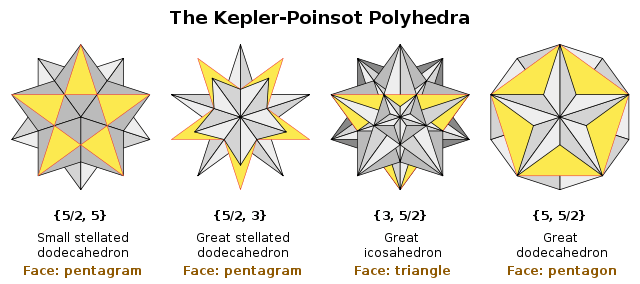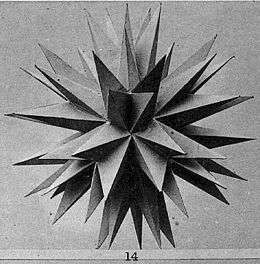Brückner's model(Taf. XI, Fig. 14, 1900)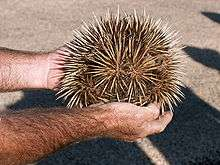The echidna

## Interpretations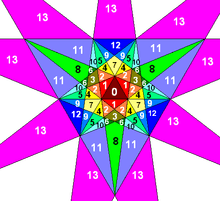Stellation diagram of the icosahedron with numbered cells. The complete icosahedron is formed from all the cells in the stellation, but only the outermost regions, labelled "13" in the diagram, are visible.

### As a stellation

The stellation of a polyhedron extends the faces of a polyhedron into infinite planes and generates a new polyhedron that is bounded by these planes as faces and the intersections of these planes as edges. The Fifty Nine Icosahedra enumerates the stellations of the regular icosahedron, according to a set of rules put forward by J. C. P. Miller, including the complete stellation. The Du Val symbol of the complete stellation is H, because it includes all cells in the stellation diagram up to and including the outermost "h" layer.

### As a simple polyhedron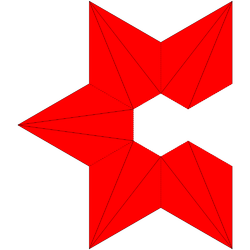A polyhedral model can be constructed by 12 sets of faces, each folded into a group of five pyramids.

As a simple, visible surface polyhedron, the outward form of the final stellation is composed of 180 triangular faces, which are the outermost triangular regions in the stellation diagram. These join along 270 edges, which in turn meet at 92 vertices, with an Euler characteristic of 2.

The 92 vertices lie on the surfaces of three concentric spheres. The innermost group of 20 vertices form the vertices of a regular dodecahedron; the next layer of 12 form the vertices of a regular icosahedron; and the outer layer of 60 form the vertices of a nonuniform truncated icosahedron. The radii of these spheres are in the ratioConvex hulls of each sphere of vertices
Inner Middle Outer All three
20 vertices 12 vertices 60 vertices 92 vertices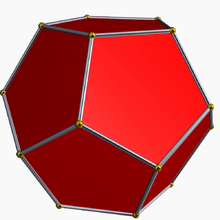DodecahedronIcosahedronNonuniform
truncated icosahedronComplete icosahedron

When regarded as a three-dimensional solid object with edge lengths a, φa, φ2a and φ2a√2 (where φ is the golden ratio) the complete icosahedron has surface areaand volume### As a star polyhedron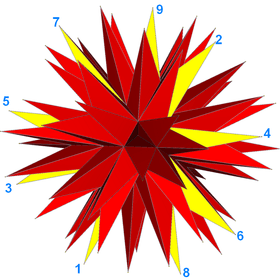Twenty (9/4) polygon faces (one face is drawn yellow with 9 vertices labeled.)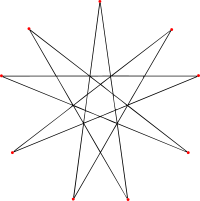2-isogonal (9/4) faces

The complete stellation can also be seen as a self-intersecting star polyhedron having 20 faces corresponding to the 20 faces of the underlying icosahedron. Each face is an irregular 9/4 star polygon, or enneagram. Since three faces meet at each vertex it has 20 × 9 / 3 = 60 vertices (these are the outermost layer of visible vertices and form the tips of the "spines") and 20 × 9 / 2 = 90 edges (each edge of the star polyhedron includes and connects two of the 180 visible edges).

When regarded as a star icosahedron, the complete stellation is a noble polyhedron, because it is both isohedral (face-transitive) and isogonal (vertex-transitive).

## Notes

1. Coxeter et al. (1938), pp 30–31
2. Wenninger, Polyhedron Models, p. 65.
3. Brückner, Max (1900)
4. Louis Poinsot, Memoire sur les polygones et polyèdres. J. de l'École Polytechnique 9, pp. 16–48, 1810.
5. Cromwell (1999) (p. 259)
6. Wheeler (1924)
7. The name echidnahedron may be credited to Andrew Hume, developer of the netlib polyhedron database:
"... and some odd solids including the echidnahedron (my name; its actually the final stellation of the icosahedron)." geometry.research; "polyhedra database"; August 30, 1995, 12:00 am.
8. Echidnahedron at polyhedra.org

## References

• Brückner, Max (1900). Vielecke und Vielflache: Theorie und Geschichte. Leipzig: B.G. Treubner. ISBN 978-1-4181-6590-1. (German) WorldCat English: Polygons and Polyhedra: Theory and History. Photographs of models: Tafel VIII (Plate VIII), etc. High res. scans.
• A. H. Wheeler, Certain forms of the icosahedron and a method for deriving and designating higher polyhedra, Proc. Internat. Math. Congress, Toronto, 1924, Vol. 1, pp 701–708
• H.S.M. Coxeter, Regular Polytopes, (3rd edition, 1973), Dover edition, ISBN 0-486-61480-8, 3.6 6.2 Stellating the Platonic solids, pp. 96–104
• Coxeter, Harold Scott MacDonald; Du Val, P.; Flather, H. T.; Petrie, J. F. (1999), The fifty-nine icosahedra (3rd ed.), Tarquin, ISBN 978-1-899618-32-3, MR 676126 (1st Edn University of Toronto (1938))
• Wenninger, Magnus J., Polyhedron models; Cambridge University Press, 1st Edn (1983), Ppbk (2003). ISBN 978-0-521-09859-5. (Model 42, p 65, Final stellation of the icosahedron)
• Cromwell, Peter R. (1997). Polyhedra. Cambridge University Press. ISBN 0-521-66405-5.
• Jenkins, Gerald, and Magdalen Bear. The Final Stellation of the Icosahedron: An Advanced Mathematical Model to Cut Out and Glue Together. Norfolk, England: Tarquin Publications, 1985. ISBN 978-0-906212-48-6.

(Convex) icosahedron Small triambic icosahedron Medial triambic icosahedron Great triambic icosahedron Compound of five octahedra Compound of five tetrahedra Compound of ten tetrahedra Great icosahedron Excavated dodecahedron Notable stellations of the icosahedron Regular Uniform duals Regular compounds Regular star Others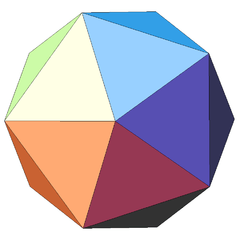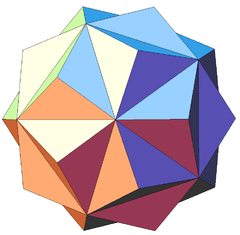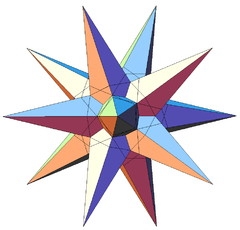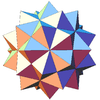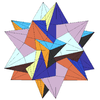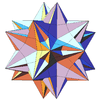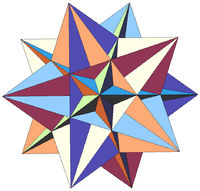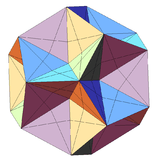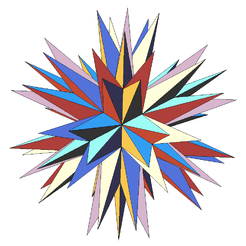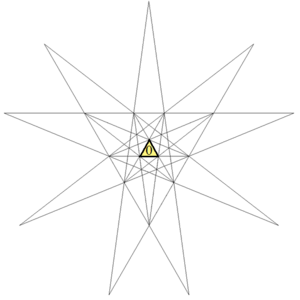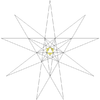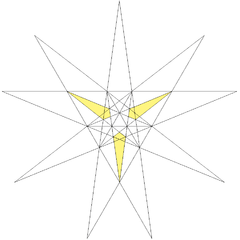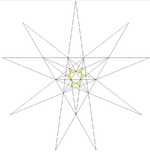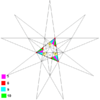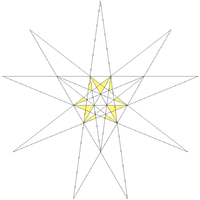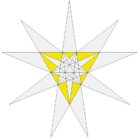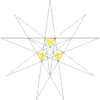The stellation process on the icosahedron creates a number of related polyhedra and compounds with icosahedral symmetry.